Verify Mean Value Theorem

Chapter 5 Class 12 Continuity and Differentiability
Concept wise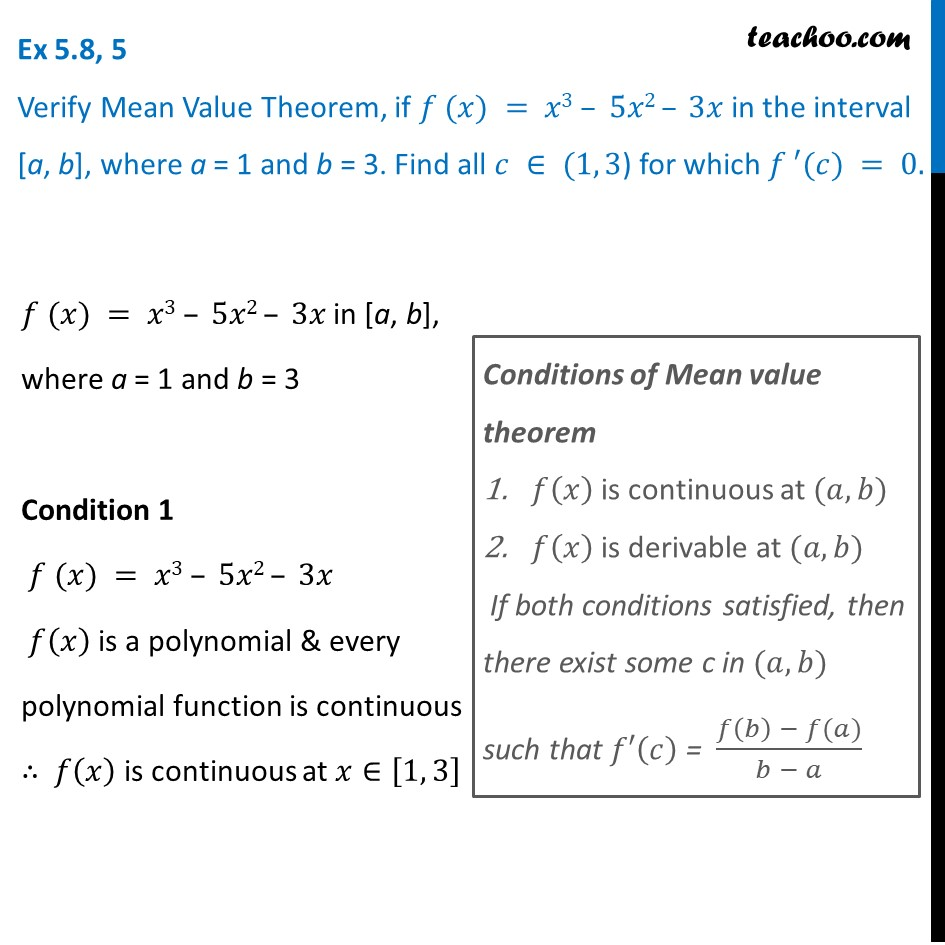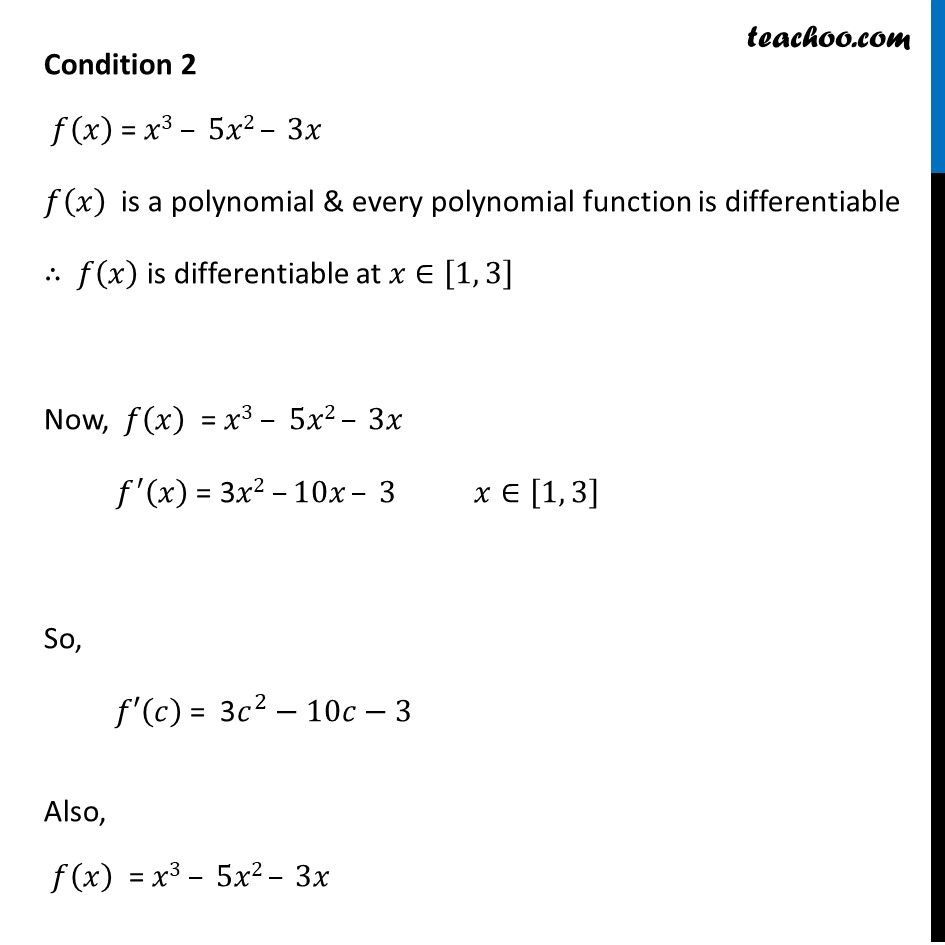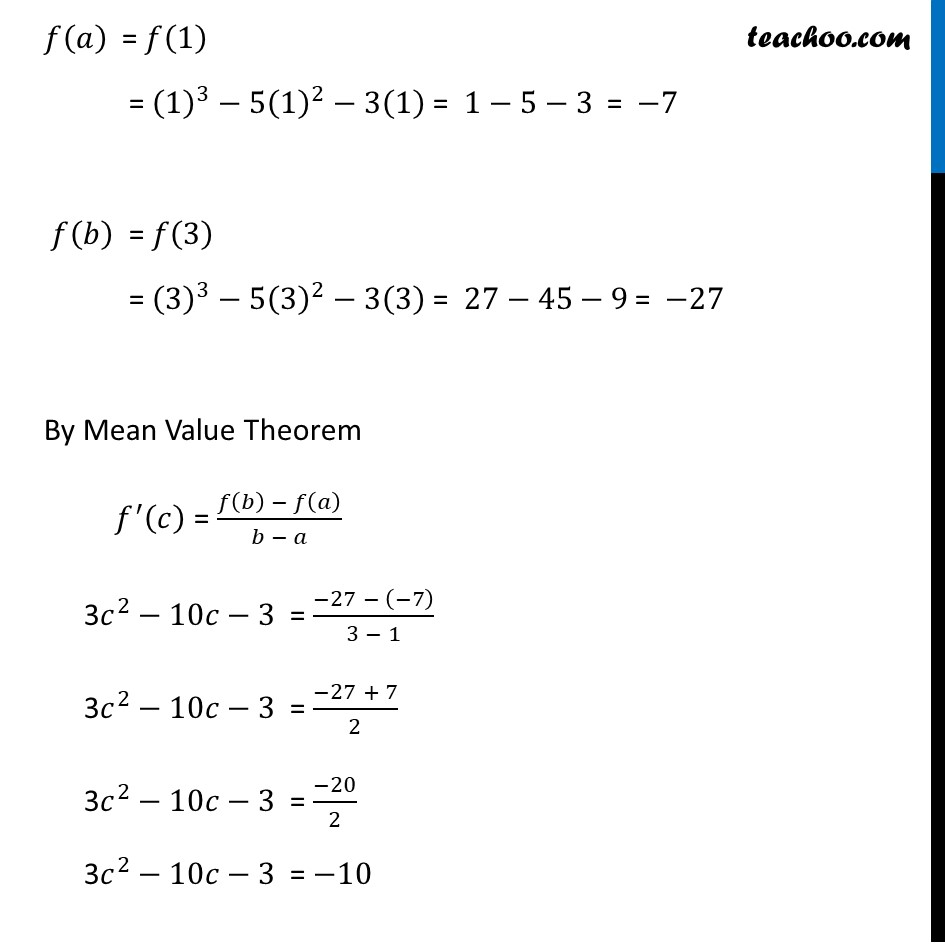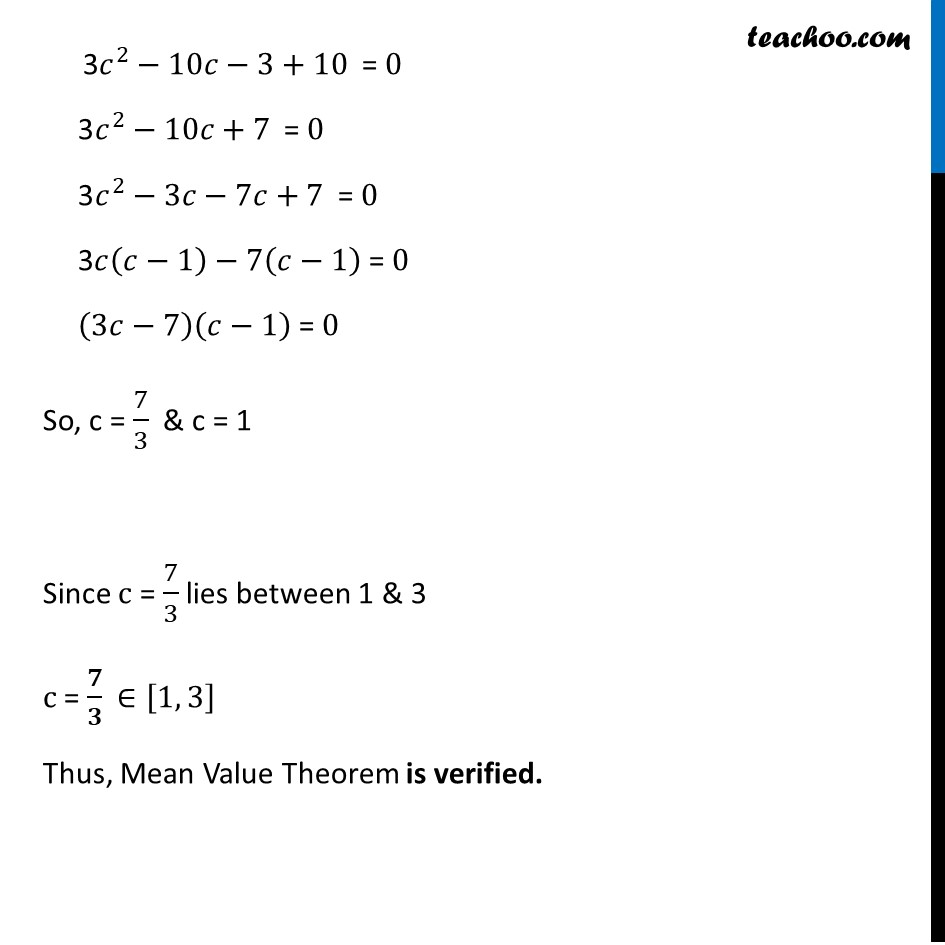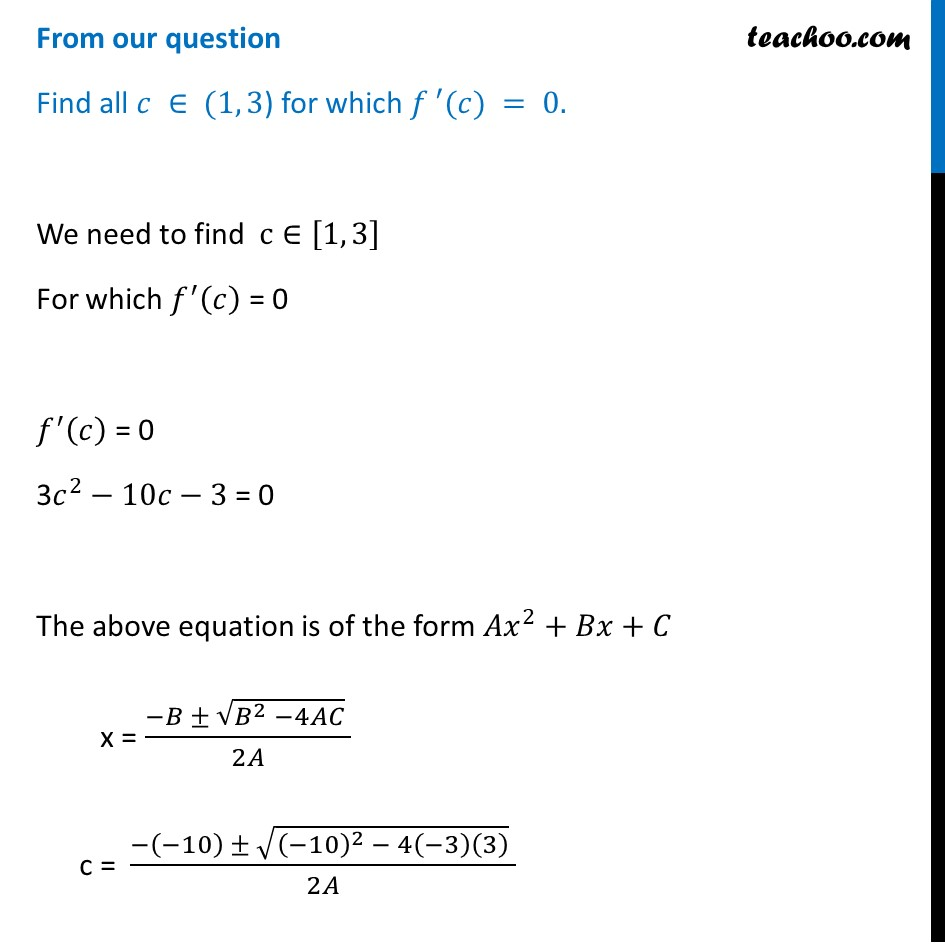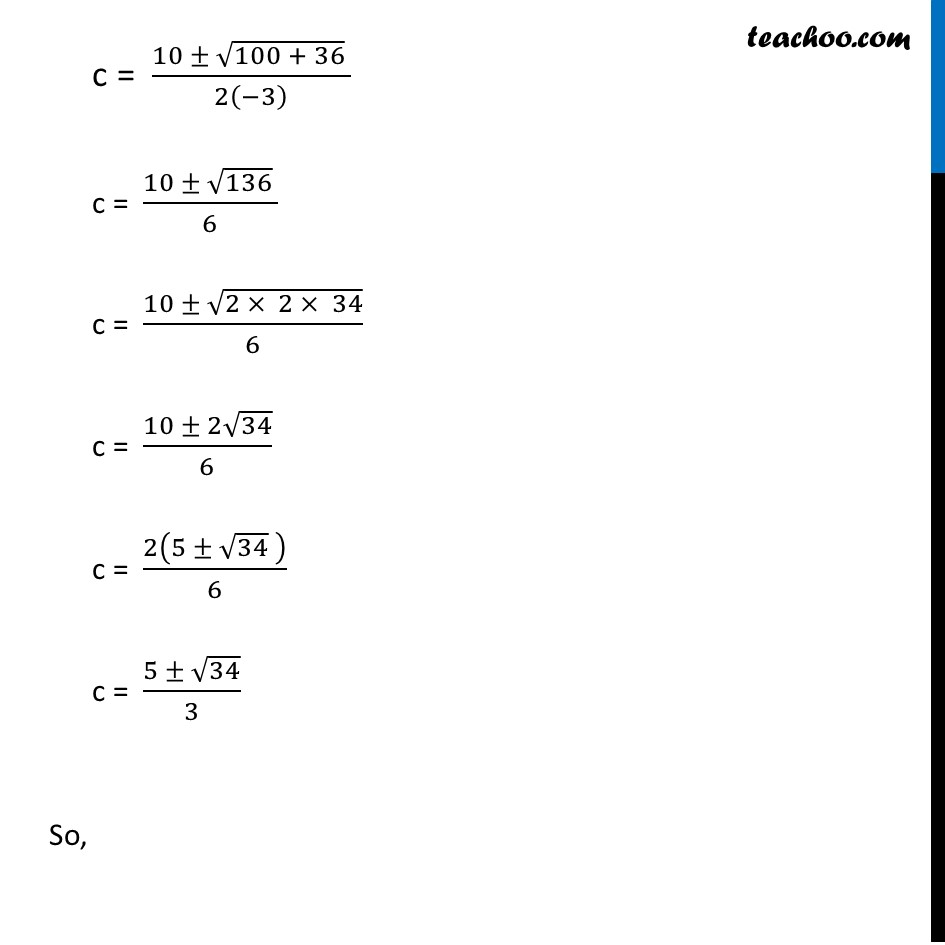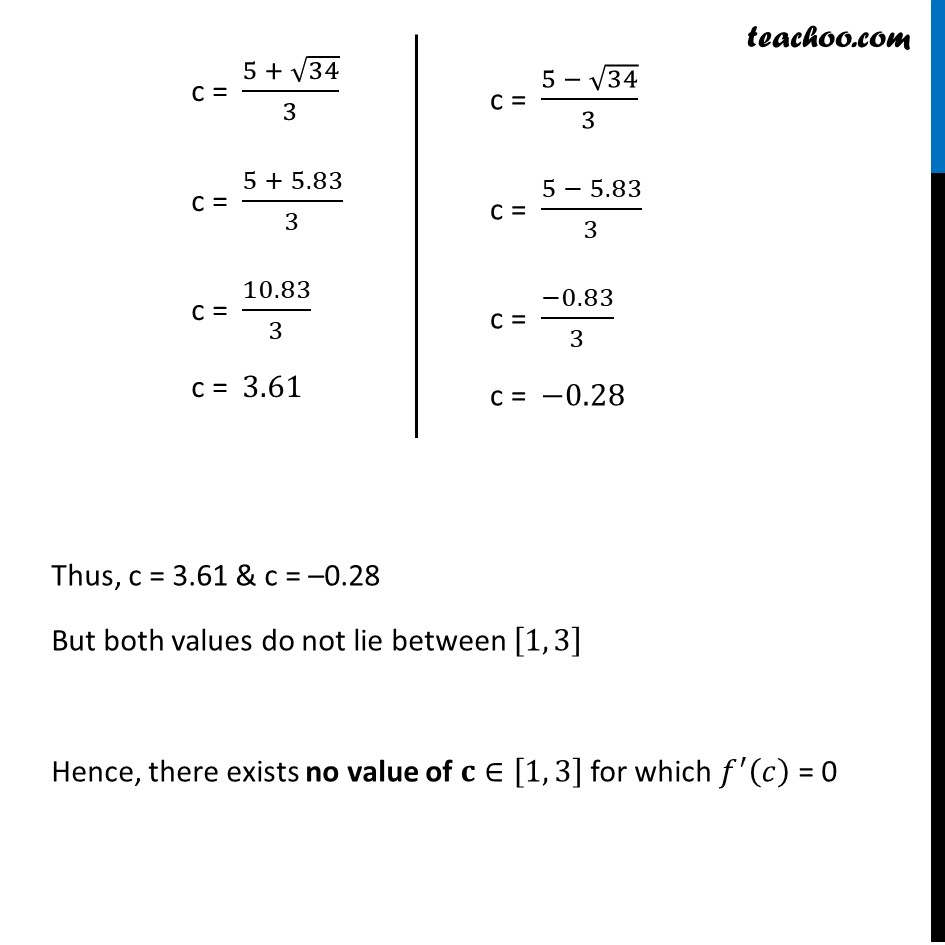Introducing your new favourite teacher - Teachoo Black, at only ₹83 per month

### Transcript

Ex 5.8, 5 Verify Mean Value Theorem, if 𝑓 (𝑥) = 𝑥3 – 5𝑥2 – 3𝑥 in the interval [a, b], where a = 1 and b = 3. Find all 𝑐 ∈ (1, 3) for which 𝑓 ′(𝑐) = 0.𝑓 (𝑥) = 𝑥3 – 5𝑥2 – 3𝑥 in [a, b], where a = 1 and b = 3 Condition 1 𝑓 (𝑥) = 𝑥3 – 5𝑥2 – 3𝑥 𝑓(𝑥) is a polynomial & every polynomial function is continuous ∴ 𝑓(𝑥) is continuous at 𝑥∈[1, 3] Conditions of Mean value theorem 𝑓(𝑥) is continuous at (𝑎, 𝑏) 𝑓(𝑥) is derivable at (𝑎, 𝑏) If both conditions satisfied, then there exist some c in (𝑎, 𝑏) such that 𝑓′(𝑐) = (𝑓(𝑏) − 𝑓(𝑎))/(𝑏 − 𝑎) Condition 2 𝑓(𝑥) = 𝑥3 – 5𝑥2 – 3𝑥 𝑓(𝑥) is a polynomial & every polynomial function is differentiable ∴ 𝑓(𝑥) is differentiable at 𝑥∈[1, 3] Now, 𝑓(𝑥)" = " 𝑥3 – 5𝑥2 – 3𝑥 𝑓^′ (𝑥)" = 3" 𝑥2 –10𝑥 – 3 𝑥∈[1, 3] So, 𝑓′(𝑐) = " 3" 𝑐^2−10𝑐−3 Also, 𝑓(𝑥)" = " 𝑥3 – 5𝑥2 – 3𝑥 𝑓(𝑎)" = " 𝑓(1) = (1)^3−5(1)^2−3(1) = 1−5−3 = −7 𝑓(𝑏)" = " 𝑓(3) = (3)^3−5(3)^2−3(3) = 27−45−9 = −27 By Mean Value Theorem 𝑓^′ (𝑐) = (𝑓(𝑏) − 𝑓(𝑎))/(𝑏 − 𝑎) "3" 𝑐^2−10𝑐−3 = (−27 − (−7))/(3 − 1) "3" 𝑐^2−10𝑐−3 = (−27 + 7)/2 "3" 𝑐^2−10𝑐−3 = (−20)/2 "3" 𝑐^2−10𝑐−3 = −10 "3" 𝑐^2−10𝑐−3+10 = 0 "3" 𝑐^2−10𝑐+7 = 0 "3" 𝑐^2−3𝑐−7𝑐+7 = 0 "3" 𝑐(𝑐−1)−7(𝑐−1) = 0 (3𝑐−7)(𝑐−1) = 0 So, c = 7/3 & c = 1 Since c = 7/3 lies between 1 & 3 c = 𝟕/𝟑 ∈[1, 3] Thus, Mean Value Theorem is verified. From our question Find all 𝑐 ∈ (1, 3) for which 𝑓 ′(𝑐) = 0. We need to find c∈[1, 3] For which 𝑓^′ (𝑐) = 0 𝑓^′ (𝑐) = 0 "3" 𝑐^2−10𝑐−3 = 0 The above equation is of the form 𝐴𝑥^2+𝐵𝑥+𝐶 x = (−𝐵 ± √(𝐵^2 −4𝐴𝐶) )/2𝐴 c = (−(−10) ± √((−10)^2 − 4(−3)(3) ) )/2𝐴 c = (10 ± √(100 + 36) )/2(−3) c = (10 ± √136 )/6 c = (10 ± √(2 × 2 × 34))/6 c = (10 ± 2√34)/6 c = 2(5 ± √34 )/6 c = (5 ± √34)/3 So, c = (5 + √34)/3 c = (5 + 5.83)/3 c = 10.83/3 c = 3.61 c = (5 − √34)/3 c = (5 − 5.83)/3 c = (−0.83)/3 c = −0.28 Thus, c = 3.61 & c = –0.28 But both values do not lie between [1, 3] Hence, there exists no value of 𝐜∈[1, 3] for which 𝑓^′ (𝑐) = 0